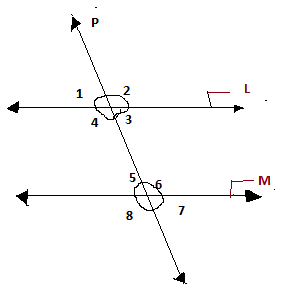# Lines and Angles:-Definition and properties

Lines and angles ,basic concepts of Geometry. Euclid (who is known as the father of geometry) described a line as “breathless length” which lies equally with respect to the points in itself. Euclid also explain plane angle as the inclination to each other in a plane of two lines which meet each other ,and do not lie straight with respect to each other. But in modern time, an angle is the figure comprised  by two rays, called the sides of the angel, sharing a common endpoint, could be the vertex of the angle.

The postulates which are based on lines are:

• Space contains at least two distinct points,
• A line contains infinitely many points and contain at least two distinct points.
• A plane is a set of many points and contains at least three non-collinear points.
• Intersection of  two distinct planes  is a straight line.
• Halves of equals is equal.
• Through a given point, there are an infinite number of lines.
• Given two distinct points A and B, there is one and only one line that contains both the points.
• Two lines can intersect each other  at most at one point.
• Two lines have having a common point are known as intersecting point.
• Two lines which are both parallel to the same line are parallel to each other.
•  Length, breadth and height cannot be measured by a dot(Point is represented by a dot).

## Brief outline of lines and angles:-

### Line segment:-

A line is said to be a line segment when it has two ends points with a fixed length or we can say a straight path between two points.

### Ray:-

A line is s aid to be Ray when it has one fixed starting point with no end points. It extends infinitely in one direction. It is exhibited by an arrow.

#### Question: How many rays are there in an angle?

Ans: There are two rays needed to make an angle.

### Straight line:-

A line that has neither initial point nor end points but extends  till infinity and has no curves is called a straight line.

Any angle can be traced by revolving line in two ways –

1.Angle formation is positive ,If the revolving line rotates in an anti-clockwise and makes an angle.

2.Angle formation is negative ,If the revolving line rotates clockwise direction to make an angle.

### What are the Types of angles ?

1: Zero angle: – The angle whose value is 0 ° is called zero angle.

2:- acute angle: – The angle whose value is greater than 0 ° and smaller than 90 °. It is called the acute angle.

3: – Right angle: – The angle whose value is 90 °. It is called a right angle.

4: – Obtuse Angle: – The angle whose value is greater than 90 ° and smaller than 180 °. It is called the obtuse angle.

5: Angular angle/Straight Angle: – The angle whose value is 180 °. It is called the angular angle.

6: – Reflex angle: – The angle whose value is greater than 180 ° and smaller than 360 °. It is called the Reflex angle.

7: – Full/Complete angle: – The angle whose value is 360 °. It is also called the complete angle.

## ANGLE TYPES

1: – Kotipurak angle or complementary angle: – If the sum of the measures of two angles is 90 °, then they are called complimentary  angle.

2: -Supplementary angle: – If the sum of measures of two angles is 180 °, then they are called Supplementary angles .When two angles are supplementary ,each angle is said to be Supplement of the other.

i-if they have a common vertex,

ii-if they  have a common arm,

iii- the common arms are on either side of the common arm  .

Adjacent angles have a common vertex and a common arm but do not overlap.

4:-Linear pair :–  A linear pair is a pair of adjacent angles whose non-common sides are opposite rays.

Vertically Opposite Angle:

When two lines intersect,4 angles are formed and the angles opposite to each other are known as vertically opposite angles and they are equal.## Lines and Angles:-Definition and properties

∠1, ∠2, ∠3, and ∠4 are formed. vertically opposite angles are

∠1 and ∠3, ∠2 and ∠4.

∠1=∠3

∠2=∠4.

### Angles  made by a transversal:### Angles  made by a transversal:

Two lines L and M cut by transversal P. The 8 angles formed have special names

 Angles name Which angles Interior angles ∠3, ∠4, ∠5, ∠6 Exterior angles ∠1, ∠2, ∠7, ∠8 Pairs of corresponding angles ∠1 and ∠5, ∠2 and ∠6, ∠4 and ∠8, ∠3 and ∠7 Pairs of alternate interior angles ∠4 and ∠6, ∠3 and ∠5 Pairs of alternate exterior angles ∠1 and ∠7, ∠2 and ∠8

### Transversal of parallel lines:Lines and Angles:-Definition and properties
• If two parallel lines are cut by another line i.e. transversal,8 angles are formed and each pair of corresponding angles are equal in measures.
• If two parallel lines are cut by another line i.e. transversal,8 angles are formed and each pair of alternate interior angles are equal.
• If two parallel lines are cut by another line i.e. transversal,8 angles are formed and each pair of interior angles on the same side of transversal are supplementary.
Solved Questions:

#### 1. How many acute angles are there in letter ‘V’.

Answer- There is only one angle and that is an acute angle available in letter ‘V’.

### Ans-

·        Acute
·        Obtuse
·        Right
·        Straight
·
Reflex

Ans.180°

### 3.what is zero angle?

Ans-An angle whose measure is equal to zero degree.it is very difficult to visualize it.